## CVR using breath-hold

The test data for this tutorial consists of a pre-processed BOLD dataset acquired using a 7 tesla Philips MRI system. The volunteer was instructed to perform 3 consecutive breath-hold periods in order to evoke a CVR response. Simply unzip the test data file and update the data path (/Data) pointing to the image files along with the sequence path (/Data/traces) in the Matlab demo file.

TUTORIAL 2: BOLD-CVR based on breath-hold stimulus

# TUTORIAL 2: BOLD-CVR based on breath-hold stimulus

This tutorial comprises a template data analysis script that utilizes native matlab functions as well as functions from the seeVR toolbox. If you use any part of this process or functions from this toolbox in your work please cite the following article:
Champagne AA, Bhogal AA., Insights Into Cerebral Tissue-Specific Response to Respiratory Challenges at 7T: Evidence for Combined Blood Flow and CO2-Mediated Effects. Front Physiol. 2021 Jan 28;12:601369. doi: 10.3389/fphys.2021.601369. PMID: 33584344; PMCID: PMC7876301.
If you have suggestions for improvement, please contact me at a.bhogal@umcutrecht.nl, https://www.seevr.nl/contact-collaboration/ or feel free to start a new branch at https://github.com/abhogal-lab/seeVR
************************************************************************************************************************************
meanTimeseries: calculates the average time-series signal intensity in a specific ROI
demeanData: subtracts the mean value from each voxel time-series
normTimeseries: normalizes time-series data to a specified baseline period
remLV: generates a mask that can be used to isolate and remove large vessel signal contributions
scrubData: uses nuisance regressors to remove unwanted signal contributions
smthData: perfomes gaussian smoothing operation on image/time-series data
glmCVRidx: uses a general linear model approach to calculate a 'CVR index' - suitable when CO2 data is not available
lagCVR: calculates CVR and hemodynamic lag using a cross-correlation or lagged-GLM approach

### MRI Data Properties

This example uses BOLD data acquired using a Philips 7 tesla MRI scanner with the
following parameters:
Scan resolution (x, y): 112 109
Scan mode: MS
Repetition time [ms]: 1700
Echo time [ms]: 25
FOV (ap,fh,rl) [mm]: 224 59.2 224
Scan Duration [sec]: 281
Slices: 27
Volumes: 160
EPI factor: 37
Slice thickness: 2mm
Slice gap: 0.2mm
In-place resolution (x, y): 2mm

### MRI Data Processing

Simple pre-processing of MRI data was done using FSL (https://fsl.fmrib.ox.ac.uk/fsl/fslwiki/) as follows
1) motion correction (MCFLIRT)
2) calculate mean image (FSLMATHS -Tmean)
3) brain extraction (BET f = 0.2 -m)
4) tissue segmentation on BOLD image (FAST -t 2 -n 3 -H 0.2 -I 6 -l 20.0 -g --nopve)

# Analysis Pipeline

### 1) Setup options structure and load data

The seeVR functions share parameters using the 'opts' structure. In the current version this is implemented as a global variable (subect to change in the future). To get started, first initialize the global opts struct. Nifti data can be loaded using the loadImageData wrapper function. This function will also initialize certain variables in the opts structure including opts.TR (repetition time). opts.dyn (number of volumes), opts.voxelsize (resolution), opts.xdata (time vector for plotting etc.), and opts.headers (used by saveImageData to save timeseries, parameter maps and masks in opts.headers.ts/map/mask). If you use your own functions to load your imaging data, ensure that you also fill variables in the opts structure to ensure maintained functionality. If functions throw errors, it is usually because a necessary option is not specified before the function call (see manual).
clear all
clear global all
close all
% initialize the opts structure (!)
global opts
opts.verbose = 0;
% Set the location for the MRI data
cd(dir)
file = 'BOLD_mean_brain_seg_0.nii.gz';
file = 'BOLD_mean_brain_seg_1.nii.gz';
file = 'BOLD_mean_brain_seg_2.nii.gz';

### 2) Setup directories for saving output

Pay special attention to the savedir, resultsdir and figdir as you can use these to organize the various script outputs.
% specify the root directory to save results
opts.savedir = dir;
% specify a sub directory to save parameter maps - this
% directory can be changed for multiple runs etc.
if ispc
opts.resultsdir = [dir,'RESULTS\']; mkdir(opts.resultsdir);
else
opts.resultsdir = [dir,'RESULTS/']; mkdir(opts.resultsdir);
end
% specify a sub directory to save certain figures - this
% directory can be changed for multiple runs etc.
if ispc
opts.figdir = [dir,'FIGURES\']; mkdir(opts.figdir);
else
opts.figdir = [dir,'FIGURES/']; mkdir(opts.figdir);
end

### 3) Take a quick look at the data

Use the 'meanTimeseries' function with any mask to look at the ROI timeseries. To see the differences between GM, WM and whole-brain timeseries, use the 'demeanData' function before plotting. We can also use the 'normTimesieres' function to normalize the BOLD data and look at the %BOLD signal change relative to the baseline period. There are two ways to do this: 1) specify the baseline start and end indexes; 2) select the baseline start and end points.
figure(10);
TS1 = demeanData(TS1);
% Plot GM timeseries
plot(opts.xdata, TS1, 'k'); title('3X inspiratory breath-hold');
xlabel('absolute signal'); ylabel('time (s)'); xlim([0 opts.xdata(end)]);
% Overlay WM timeseries
hold on
TS2 = demeanData(TS2);
plot(opts.xdata, TS2, 'c');
title('3X inspiratory breath-hold'); xlim([0 opts.xdata(end)]);
% Overlay WB timeseries
TS3 = demeanData(TS3);
plot(opts.xdata, TS3, 'm');
title('3X inspiratory breath-hold'); xlim([0 opts.xdata(end)]);
legend('GM','WM', 'WB')
hold off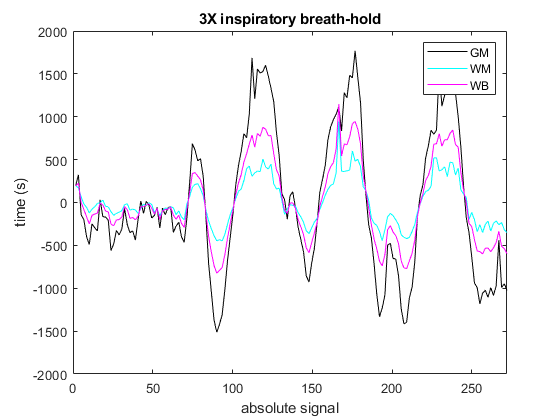clear TS1 TS2 TS3
% Normalize to baseline using interactive mode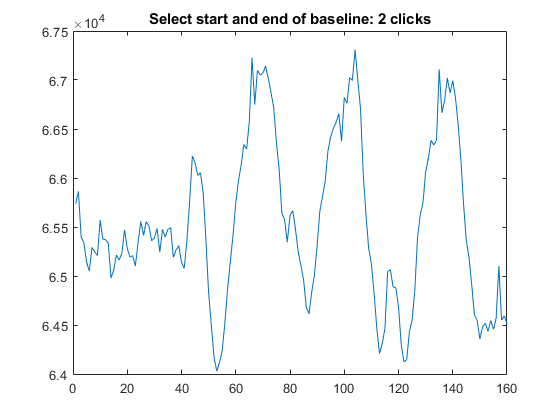% Generate mean timeseries from normalized data
% Plot normalized timeseries
figure;
plot(opts.xdata, nTS, 'k');
title('normalized GM timeseries');
ylabel('% signal change'); xlabel('time (s)'); xlim([0 opts.xdata(end)]);
% Alternatively, specify indices; this can be useful when looping over data
% or repeating analysis. The 'chopTimeseries' function works the same.
clear nTS1 nTS
index = [5 15];
hold on
plot(opts.xdata, nTS, 'm');
legend('manual', 'automatic')
set(gcf, 'Units', 'pixels', 'Position', [200, 500, 600, 160]);
hold off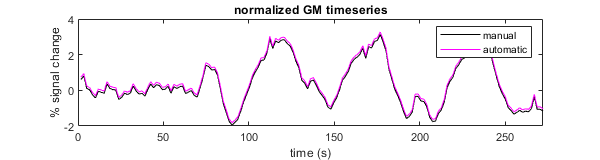clear nTS1 nTS

### 4) Regress out motion parameters

Often, breath-hold data can be somewhat corrupted due to motion artefacts. Imagine a subect is instructed to do an inspiratory BH (considered more tolerable than expiratory BH), when they inhale, the lungs expand and this can lead to tilting of the head. This motion can lead to artefacts in our BOLD signal that correlate with our BH task. We can examine the motion parameters and see if it is worthwhile to regress them out from our BOLD data. One of the strengths of the seeVR functions is the ability to play with explanatory regressors and nuissance regressors. FSL's MCFLIRT function was used for re-alignment and we can load the motion parameters as nuisance regressors. Alternate registration tools may output motion parameters in a different format but usually it is a simple text file. To regress out motion parameters, use the 'scrubData' function. This function accepts an array or nuisance regressors and data probes. Normaly the data probe would be an end-tidal gas trace, however since we do not have a trace in this example, simply use the whole brain timeseries (de-meaned and rescaled).
cd(dir) % Go to our data directory
mpfilename = 'BOLD_mcf.par'; % Find MCFLIRT motion parameter file
% Calculate motion derivatives
dtnuisance = gradient(nuisance); % temporal derivative
sqnuisance = nuisance.*nuisance; % square of motion
motion = [nuisance dtnuisance sqnuisance];
% Demean/rescale regressors
for ii=1:(size(motion,2)); motion(:,ii) = demeanData(rescale(motion(:,ii))); end
% Plot
figure; plot(opts.xdata, motion);
title('motion parameters & derivatives'); xlabel('time(s)');
xlim([0 length(motion)]);
set(gcf, 'Units', 'pixels', 'Position', [200, 500, 600, 260]);
% Scrub data
[cleanData] = scrubData(BOLD,WBmask, [nuisance dtnuisance sqnuisance], data_probe, opts);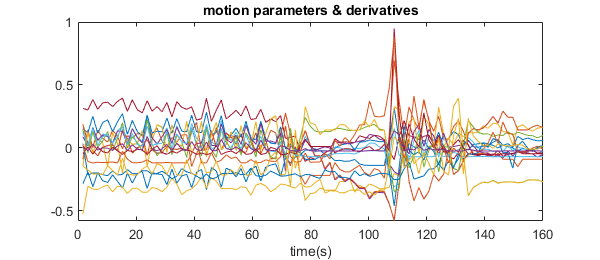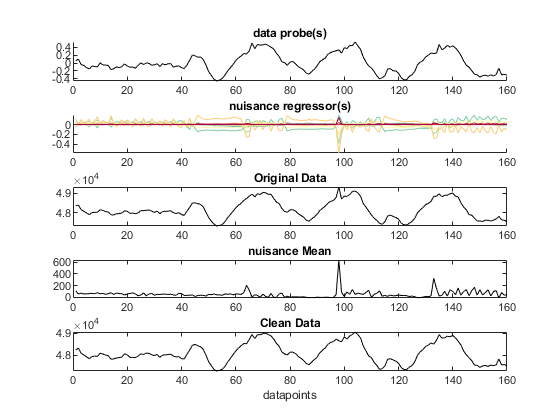% plot
figure;
hold on
legend('before scrubbing', 'after scrubbing')
hold off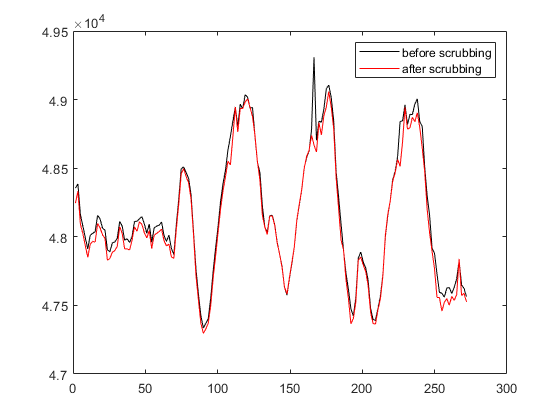As you can see, a large spike artefact has been removed. When considering things like lag analysis, such stationary spike artefacts can artificially pull correlations, thus obscuring underlying hemodynamic delays. Regression of nuisance parameters can have more or less effects depending on the data. For this reason it is important to consider that a 'one-size-fits-all' approach may not always work. Regressors can be filtered using the 'filtRegressor' function that seperates input regressors based on how well they correlate with a signal of interest (usually BOLD timeseries). The correlation threshold is defined using the opts.motioncorr parameter (see TUTORIAL 1). You can also consider to add resampled (to TR) physiological traces (HR or respiratory) as well as Legendre polynomials etc...
% First specify which order of legendre polynomials you wish to use.
opts.legOrder = [1 2 3]; %first through third order
for ii=opts.legOrder
L(ii,:) = LegendreN(ii,opts.xdata);
end
% now you can add these along with the others:
nuissance_regressors = [nuisance dtnuisance sqnuisance L'];

### 5) Removing contributions from large vessels

CVR data can often be weighted by contributions from large vessels that may overshadow signals of interest. Particulary, for very high resolution acquisitions at high field strength. We can modify the whole brain mask to exclude CSF and large vessel contributions using the 'remLV' function ('remove large vessels'). This can be done by specifying the percentile threshold from which to remove voxels, or if the appropriate toolbox is not available, a manual threshold value.
% Supply necessary options
% Define the cutoff percentile (higher values are removed; default = 98);
opts.LVpercentile = 97;
% If the stats toolbox is not present, then a manual threshold must
% be applied. This can vary depending on the data (i.e. trial and error).
% Plot
figure;
z = 8;
subplot(1,3,1)
imagesc(imrotate(mean(BOLD(:,:,z,:),4),90));
title('mean image', 'Color', 'w'); colormap(gray); cleanPlot;
subplot(1,3,2)
subplot(1,3,3)
set(gcf, 'Units', 'pixels', 'Position', [200, 500, 600, 160]);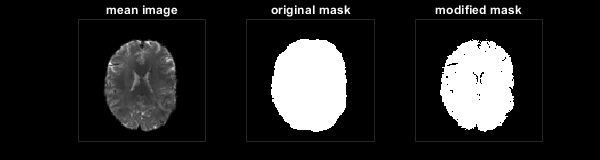### 6) Calculating CVR

In a perfect world we would have some idea of arterial gas tensions to which we could relate our BOLD signal changes. This would allow us to normalized our data and express signal changes as a function of changes in mmHg of CO2 or O2. For simple BH experiments this is often not the case - due to the need for gas monitoring systems etc. In cases where gas traces are not available, CVR maps can be generated using the 'glmCVRidx' function. This functions uses a reference signal (i.e. from a GM or cerebelum mask) as a explanatory variable and outputs a parameter map of beta values that can be interpreted as the relative CVR response. Signals are first band-pass filtered using the frequency range specified in the opts.fpass parameter. The function returns the CVRidx map, the band passed reference signal used for normalization and the band-passed BOLD timeseries if specified. Resulting maps are saved in opts.resultsdir\CVRidx\. NOTE this function can also be used to estimate CVR from resting state fMRI.
% Normalize the cleaned BOLD data (step 4) to baseline
nBOLD = normTimeseries(cleanData, mWBmask, [5 25]);
opts.fpass = [0.0001 0.2]; % accept a wide frequency band (for LFO you can set this to 0.0001 --> 0.08 for example
opts.normalizeCVRidx = 1; %this is the default. If set to zero, beta values are not normalized to the reference response
opts.smoothmap = 1; % since our BOLD data is so far not smoothed, set this to '1' to smooth output maps (default = 0)
opts.spatialdim = 3; % specify 3D gaussian smoothing (default 2D smoothing)
opts.FWHM = 3; % specify FWHM of smoothing kernel (default 4mm)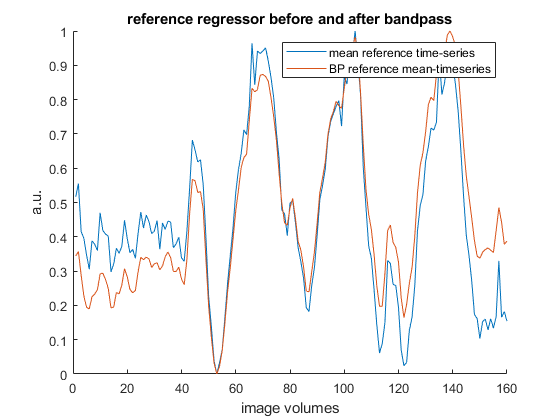% Plot
figure;
colormap(flip(brewermap(256,'Spectral'))); % for schemes see /seeVR/plotting/colormaps/brewer/maps.png
step = 5;
z=4;
scl = [0 2];
for ii=0:step-1
subplot(1,5,ii+1)
imagesc(imrotate(CVRidx(:,:,z + ii*step),90), scl); freezeColors;
cleanPlot; if ii == 0; ylabel('CVR', 'color', 'w'); end
end
set(gcf, 'Units', 'pixels', 'Position', [10, 100, 1000, 160]);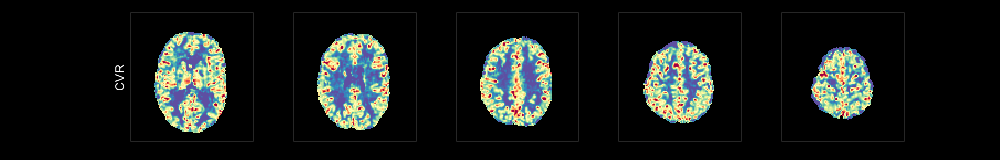clear nBOLD

### 7) Calculating Hemodynamic Lag

In addition to CVR, hemodynamic lag can be another interesting parameter to consider when looking at vascular impairment. A common approach to doing this involves performing a cross-correlation or lagged-GLM approach with some stimulus waveform (PetCO2 or PetO2). The calculated lags with respect to this waveform probe are then plotted voxel-wise to produce a lag parameter map. A more advanced approach is to use this input probe in order to generate an optimized regressor (see: https://rapidtide.readthedocs.io/en/stable/). This analysis technique has also been implemented in the seeVR toolbox in the 'lagCVR' function. This function is capable of generating an optimized BOLD regressor, lag maps based on both a cross-correlation and/or lagged-GLM analysis, CVR maps as well as many statistical maps to evaluate analysis outputs. Since we do not have any breathing traces in this example, we will use the signal as a probe through which to select highly correlated voxels that will comprise the optimized BOLD regressor. Furthermore, we will skip generating CVR maps (lagCVR performs linear regression of the BOLD signal against the end-tidal trace to generate CVR/O2-resonse maps) - we've anyways already created the CVR index maps in step 6.
% First lets smooth our cleaned BOLD (step 4) data using the same smoothing
% parameters applied in step 6. We're using a lot of smoothing mostly for
% visual purposes - the SNR of 7T BOLD data is generally quite high. Use
% the modified WBmask from step 5 to avoid some of the larger vessels.
opts.spatialdim = 3; % specify 3D gaussian smoothing (default 2D smoothing)
opts.FWHM = 3; % specify FWHM of smoothing kernel (default 4mm)
% Normalize the smoothed data to the baseline period
nBOLD = normTimeseries(sBOLD, mWBmask, [5 25]);
% The lagCVR function has many options and possible outputs; some of which
% more useful than others. For more details see the seeVR manual.
% Setup main probe: here we will use the rescaled GM signal from the
% smoothed and normalized BOLD timeseries. We multiply the GM mask with the
% modified WBmask to remove any large vessel contributions
% lagCVR has the option to add a nuissance regressor (for example a PetCO2
% trace when focusing on using PetO2 as the main explanatory regressor).
% This regressor is only used when opts.glm_model = 1 (default = 0). For this example we
% can supply an empty variable since we won't use the lagged-glm approach.
nuisance = [];
% Since we are using an input probe generated from the BOLD data, we will
% only output results using the optimized regressor. In cases where we have
% the CO2 traces, we may consider to also do a straight correlation with
% the PetCO2 trace or an HRF-convolved trace (se 'convHRF' function).
opts.trace_corr = 0; %default = 1
% Since we dont have a CO2 trace and already produced maps in step 6
opts.cvr_maps = 0; %default = 1
% Produces the optimized regressor (default = 1). When this is set to 0; a straight correlation
% with the input probe is done
opts.refine_regressor = 1; %default = 1
% Factor by which to temporally interpolate data. Better for picking up
% lags between TR. Higher value means longer processing time and more RAM
% used (so be careful)
opts.interp_factor = 5; %default is 4
% The correlation threshold is an important parameter for generating the
% optimized regressor. For noisy data, a too low value will throw an error.
% Ideally this should be set as high as possible, but may need some trial
% and error.
opts.corrthresh = 0.6; %default is 0.7
% Tthresholds for refinening optimized regressors. If you make this range too large
% it smears out your regressor and lag resolution is lost. When using a CO2
% probe, the initial 'bulk' alignment becomes important here as well. A bad
% alignment will mean this range is also maybe not appropriate or should be
% widened for best results. Since we use the actual BOLD as a probe we keep
% this range tight. (ASSUMING TR ~1s!, careful).
opts.lowerlagthresh = -1; %default is -3
opts.upperlagthresh = 1; %default is 3
% Lag thresholds for lag map creation. Since we are looking at a healthy
% brain, this can be limited to between 20-60TRs. For impariment you can consider
% to raise the opper threshold to between 60-90TRs. (ASSUMING TR ~1s!, careful).
opts.lowlag = -5; %setup lower lag limit; negative for misalignment and noisy correlation
opts.highlag = 20; %setups upper lag limit; allow for long lags associated with pathology
% For creating the optimized BOLD regressor, we will only use GM voxels for
% maximum CO2 sensitivity. We will also remove large vessel contributions.
%remove CSF from analysis
Perform hemodyamic analysis
probename = 'final_probe.mat'
Creating optimized regressor Correlating TS voxelwise to probe 1 . . . finished correlation, estimating new probe
rmse = 0.1300
Correlating TS voxelwise to probe 2 . . . finished correlation, estimating new probe
rmse = 0.0037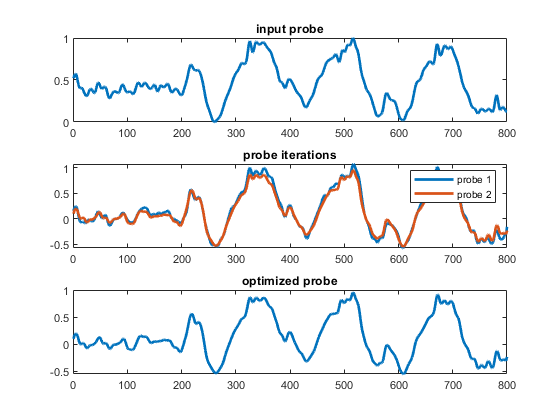Finished creating optimized regressor performing correlation based lag analysis using optimized regressor Lag, regressor and r_maps were created in: 2 minutes
% The advantage of using the global opts struct is that the variables used
% for a particular processing run (including all defaults set within
% functions themselves) can be saved to compare between runs.
save([opts.resultsdir,'processing_options.mat'], 'opts');

### 10) Plot Results

In the maps struct, we have a subfield called 'XCORR' where the results of the cross-correlation based lag results are returned. Along with using the optimized BOLD regressor, in this case we also performed a straight correlation with the input proble (this can be turned off using opts.trace_corr = 0). When both the optimized regressor and input regressor are used, the maximum r value compared between each analysis approach is used to define the lag. This is useful if one regressor is more accurate than the other for a particular voxel. Generally though, the optimized regressor (lag_opti) provides the best results.
% Plot resulting lag map with CVR maps
maps.XCORR.lag_opti(maps.XCORR.lag_opti == 0) = NaN;
maps.XCORR.r_opti(maps.XCORR.r_opti == 0) = NaN;
figure;
step = 5;
z=4;
scl = [0 2];
scllag = [2 14];
sclr = [-1 1];
for ii=0:step-1
% BOLD
subplot(4,5,ii+1)
imagesc(imrotate(BOLD(:,:,z + ii*step,1),90));
colormap(gray)
freezeColors;
cleanPlot; if ii == 0; ylabel('BOLD', 'color', 'w'); end
%CVR
subplot(4,5,(ii+1+step))
imagesc(imrotate(CVRidx(:,:,z + ii*step),90), scl);
colormap(flip(brewermap(256,'Spectral')));
freezeColors;
cleanPlot; if ii == 0; ylabel('CVR', 'color', 'w');
xlabel('CVR: 0 to 2', 'color', 'w'); end
%lags
subplot(4,5,(ii+1+2*step))
imagesc(imrotate(maps.XCORR.lag_opti(:,:,z + ii*step),90), scllag);
colormap(magma)
freezeColors;
cleanPlot; if ii == 0; ylabel('Lags', 'color', 'w');
xlabel('lags: 2 to 14s', 'color', 'w'); end
subplot(4,5,(ii+1+3*step))
imagesc(imrotate(maps.XCORR.r_opti(:,:,z + ii*step),90), sclr);
colormap(devon)
freezeColors;
cleanPlot; if ii == 0; ylabel('r', 'color', 'w');
xlabel('r xcorr: -1 to 1', 'color', 'w'); end
end
set(gcf, 'Units', 'pixels', 'Position', [10, 100, 1000, 640]);